• 下面小编就为大家分享一篇Java 堆排序实例(大顶堆、小顶堆)，具有很好的参考价值，希望对大家有所帮助。一起跟随小编过来看看吧
• 《大顶堆、小顶堆》 一、二叉堆的定义 二叉堆：首先是一棵二叉树，其次这棵二叉树要满足结构性质和堆序性质 结构性质：是一颗完全二叉树 堆序性质：对于树中的任意节点，要求key大于它的两个孩子，两个孩子...
本文目录
一、二叉堆的定义
结构性质堆序性质二、二叉堆的底层存储结构三、二叉堆的插入四、二叉堆的删除五、源码和测试

系列目录
《树》《树的遍历》《二叉查找树》《AVL》《红黑树（上）》《红黑树（下）》《B树》《B+树》《大顶堆、小顶堆》

一、二叉堆的定义
二叉堆：首先是一棵二叉树，其次这棵二叉树要满足结构性质和堆序性质
结构性质：是一颗完全二叉树堆序性质：
大顶堆：对于树中的任意节点，要求key大于等于它的两个孩子，两个孩子之间没有排序要求，所以根节点拥有最大值小顶堆：对于树中的任意节点，要求key小于等于它的两个孩子，两个孩子之间没有排序要求，所以根节点拥有最小值
1.结构性质
二叉堆是一颗完全二叉树。
完全二叉树：一棵深度为k的有n个结点的二叉树，对树中的结点按从上至下、从左到右的顺序进行编号，如果编号为i（1≤i≤n）的结点与满二叉树中编号为i的结点在二叉树中的位置相同，则这棵二叉树称为完全二叉树。
这个定义看了就像没看一样，我们讲点通俗易懂的。
满二叉树每一层的节点都是满的：
第零层只有根节点，所以有2^0=1个节点第一层有2^1=2个节点第二层有2^2=4个节点第三层有2^3=8个节点......
如果一棵二叉树每一层的节点都是满的，这棵树就是满二叉树。下图就是一颗满二叉树。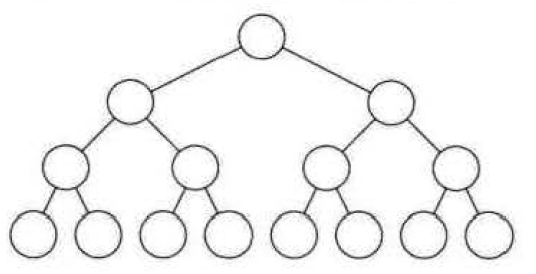满二叉树一定是一颗完全二叉树，但完全二叉树不一定是一颗满二叉树。
完全二叉树结构上很接近满二叉树，只是允许最后一层不满，并且中间不允许空洞。
下图就是一颗完全二叉树，中间没有空洞，虽然最后一层不满，但你从上到下逐层、每层从左往右的看，除了最后一层缺最后几个节点，中间一个不缺，没有一个漏洞。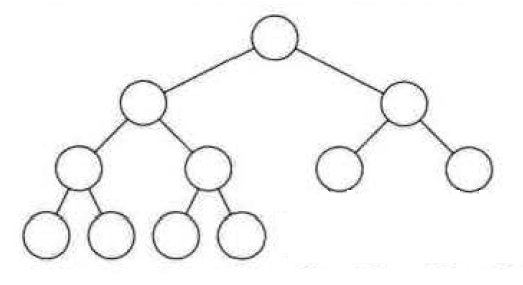像下面两图，在最后一层从左往右看的时候，你会发现中间出现了一个空洞，所以既不是满二叉树，也不是完全二叉树。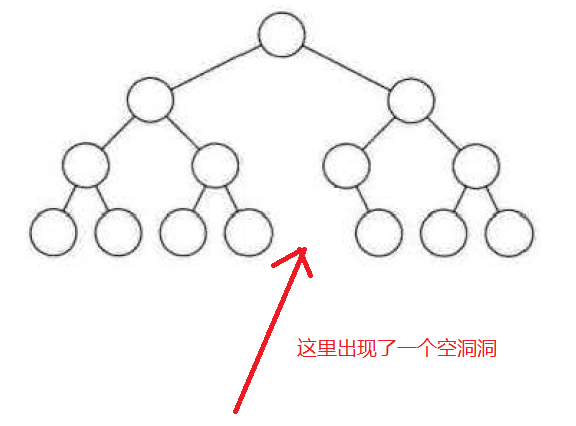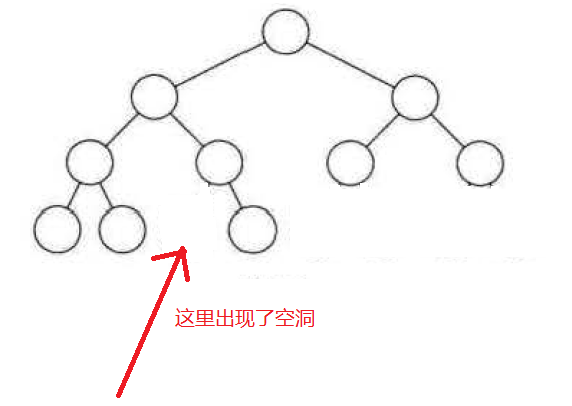2.堆序性质
如果是大顶堆，那么对于这颗完全二叉树中的任意节点，都有该节点的key大于等于它的两个孩子，而两个孩子之间没有排序的要求。
如果是小顶堆，那么对于这颗完全二叉树中的任意节点，都有该节点的key小于等于它的两个孩子，而两个孩子之间没有排序的要求。
下图就是一个小顶堆：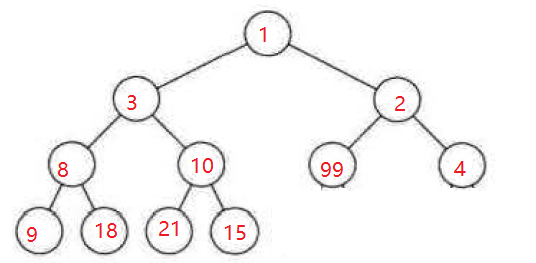这个结构虽然看起来杂乱无章，但切切实实的是一个小顶堆，稍后你看过二叉堆的源码实现你就能理解了。

二、二叉堆的底层存储结构
二叉堆的底层存储结构通常是数组，用顺序存储的数组来存放节点，并且数组的第0个下标是不用的。
所以在二叉堆中，定义一个数组用来存储插入的节点：
private Node<K, V>[] queue = new Node[size];
二叉堆的底层存储结构形如下图：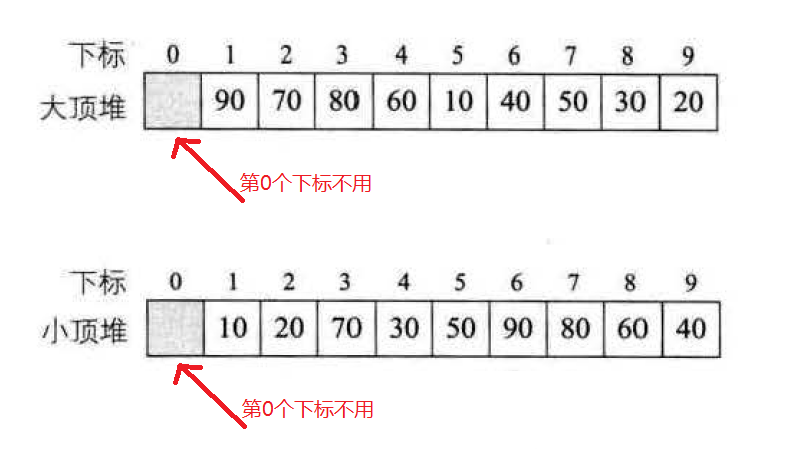第0个下标不用，这样就很容易拿到某一个节点的父节点和孩子节点。
找到某一个节点的父节点：
	/**
* 输入当前节点的index，获取父节点的index
* @param index
* @return
*/
private int parent(int index) {
return index >> 1;
}
找到某一个节点的左孩子：
	/**
* 输入当前节点的index，获取其左孩子的index
* @param index
* @return
*/
private int left(int index) {
return 1 << index;
}
找到某一个节点的右孩子：
left(index) + 1;
因为要满足完全二叉树的结构性质，所以如果某一个节点只有一个孩子，那一定是左孩子，如果是右孩子那相当于左孩子处出现空洞，也就意味着二叉堆底层存放节点的数组中间出现了值为null的下标。

三、二叉堆的插入
插入操作既不能破坏二叉堆的结构性质（完全二叉树）也不能破坏二叉堆的堆序性质。
为了不破坏二叉堆完全二叉树的结构性质，我们在二叉堆中定义一个size，有两个用处：
一则是用来记录堆中存放节点的数量；二则当插入节点的时候queue[++size]整好定位到新节点存放的位置，二叉堆中存放节点的数组queue[]中不会出现空洞；
为了满足二叉堆的堆序性质，新节点要不断的向上回溯：
如果是大顶堆，向上回溯直至根节点或其父节点大于等于自己；如果是小顶堆，向上回溯直至根节点或其父节点小于等于自己；
大顶堆插入示例代码：
	/**
* 向二叉堆中插入数据
* @param key
* @param value
*/
public void insert(K key, V value) {
if ((size + 1) == this.queue.length)
this.queue = Arrays.copyOf(queue, 2 * queue.length);
queue[++size] = new Node<K, V>(key, value);
fixup(size);
}

/**
* 新插入的节点放入数组index=size，这样不会破坏二叉堆的结构性质（完全二叉树）
* 然后拿到其父节，比较两者的key，如果新插入节点的key大于父节点的key，则两者交换位置
* 这样不断向上回溯，直至跟节点或小于其父节点的key
*/
private void fixup(int index) {
while (index > 1) {
int parentIndex = parent(index);
// 如果父亲的key大于等于自己的key，则停止向上回溯
if (queue[parentIndex].key.compareTo(queue[index].key) >= 0)
break;
// 如果父亲的key小于自己的key，则与父亲交换位置，然后继续向上回溯
Node<K, V> tmp = queue[parentIndex];
queue[parentIndex] = queue[index];
queue[index] = tmp;
index = parentIndex;
}
}

四、二叉堆的删除
二叉堆的删除操作是摘掉根节点，即把queue摘掉。
同样的，删除操作同样不能破坏二叉堆的结构性质和堆序性质。
为了保证删除操作时二叉堆完全二叉树的结构性质，当把根节点queue摘掉的时候会出现空洞，于是我们把最后一个节点queue[size]挪过来填补空洞。
为了保证删除操作时二叉堆的堆序性质，当把最后一个节点queue[size]挪到根节点的时候，我们需要不断的向下回溯：
如果是大顶堆，向下回溯直至两个孩子中最大的一个小于等于自己或已经没有孩子可以比较；如果是小顶堆，向下回溯直至两个孩子中最小的一个小于等于自己或已经没有孩子可以比较；
大顶堆删除示例代码：
	/**
* 大顶堆的根节点拥有最大值，所以deleteMax会摘掉根节点
* 这样会导致空洞，为了不破坏二叉堆完全二叉树的结构性质，将最后一个节点移动到根节点
* 然后与它的两个孩子中较大的那一个作比较，如果小于则往下移动
* 直至最后一层或大于其孩子中较大的那一个
* @return
*/
public V deleteMax() {
Node<K, V> root = queue;
queue = queue[size];
queue[size--] = null;
fixDown(1);
return root.value;
}

private void fixDown(int index) {
// 完全二叉树：如果一个节点有孩子，那一定先有左孩子
int childIndex;
/*
* 如果节点至少有一个孩子（因为是完全二叉树，所以如果有一个孩子那一定先是左孩子）
* 那么进入while循环，开始向下回溯
*/
while ((childIndex = left(index)) <= this.size) {
// 要找两个孩子中较大的那一个作比较，所以再判断一下有没有右孩子
int rightIndex = childIndex + 1;
if (rightIndex <= size && queue[rightIndex].key.compareTo(queue[childIndex].key) > 0)
childIndex = rightIndex;
// 比较较大的孩子和当前节点是否需要交换
if (queue[index].key.compareTo(queue[childIndex].key) >= 0)
break;
Node<K, V> tmp = queue[index];
queue[index] = queue[childIndex];
queue[childIndex] = tmp;
index = childIndex;
}
}

五、源码和测试
大顶堆源码：
package cn.wxy.blog2;

import java.util.Arrays;

/**
* 大顶堆
* @author 王大锤
* @date 2021年6月26日
*/
public class BinaryHeap<K extends Comparable<K>, V> {
static class Node<K extends Comparable<K>, V> {
private K key;
private V value;

public Node(K key, V value) {
this.key = key;
this.value = value;
}

public K getKey() {
return key;
}

public void setKey(K key) {
this.key = key;
}

@Override
public String toString() {
return this.key + ":" + value + " ";
}
}

@SuppressWarnings("unchecked")
private Node<K, V>[] queue = new Node;
private int size = 0;

public BinaryHeap() {
}

/**
* 输入当前节点的index，获取父节点的index
* @param index
* @return
*/
private int parent(int index) {
return index >> 1;
}

/**
* 输入当前节点的index，获取其左孩子的index
* @param index
* @return
*/
private int left(int index) {
return 1 << index;
}

/**
* 向二叉堆中插入数据
* @param key
* @param value
*/
public void insert(K key, V value) {
if ((size + 1) == this.queue.length)
this.queue = Arrays.copyOf(queue, 2 * queue.length);
queue[++size] = new Node<K, V>(key, value);
fixup(size);
}

/**
* 新插入的节点放入数组index=size，这样不会破坏二叉堆的结构性质（完全二叉树）
* 然后拿到其父节，比较两者的key，如果新插入节点的key大于父节点的key，则两者交换位置
* 这样不断向上回溯，直至跟节点或小于其父节点的key
*/
private void fixup(int index) {
while (index > 1) {
int parentIndex = parent(index);
// 如果父亲的key大于等于自己的key，则停止向上回溯
if (queue[parentIndex].key.compareTo(queue[index].key) >= 0)
break;
// 如果父亲的key小于自己的key，则与父亲交换位置，然后继续向上回溯
Node<K, V> tmp = queue[parentIndex];
queue[parentIndex] = queue[index];
queue[index] = tmp;
index = parentIndex;
}
}

/**
* 大顶堆的根节点拥有最大值，所以deleteMax会摘掉根节点
* 这样会导致空洞，为了不破坏二叉堆完全二叉树的结构性质，将最后一个节点移动到根节点
* 然后与它的两个孩子中较大的那一个作比较，如果小于则往下移动
* 直至最后一层或大于其孩子中较大的那一个
* @return
*/
public V deleteMax() {
Node<K, V> root = queue;
queue = queue[size];
queue[size--] = null;
fixDown(1);
return root.value;
}

private void fixDown(int index) {
// 完全二叉树：如果一个节点有孩子，那一定先有左孩子
int childIndex;
/*
* 如果节点至少有一个孩子（因为是完全二叉树，所以如果有一个孩子那一定先是左孩子）
* 那么进入while循环，开始向下回溯
*/
while ((childIndex = left(index)) <= this.size) {
// 要找两个孩子中较大的那一个作比较，所以再判断一下有没有右孩子
int rightIndex = childIndex + 1;
if (rightIndex <= size && queue[rightIndex].key.compareTo(queue[childIndex].key) > 0)
childIndex = rightIndex;
// 比较较大的孩子和当前节点是否需要交换
if (queue[index].key.compareTo(queue[childIndex].key) >= 0)
break;
Node<K, V> tmp = queue[index];
queue[index] = queue[childIndex];
queue[childIndex] = tmp;
index = childIndex;
}
}

/**
* 层序遍历二叉堆，实际上就是顺序打印二叉堆的底层数组
*/
public void traversalHeapByLevel() {
System.out.print("打印堆queue[]：");
for (Node<K, V> node : queue)
System.out.print(node + " ");
System.out.println();
}

/**
* 通过删除根节点来遍历大顶堆
*/
System.out.print("循环deleteMax：");
while(size > 0)
System.out.print(deleteMax() +" ");
System.out.println();
}
}
测试代码：
	public static void main(String[] args) {
int[] array = {8, 57, 11, 34, 53, 71, 87, 21, 98, 81};
printArray(array);
BinaryHeap<Integer, String> heap = new BinaryHeap<Integer, String>();
for (int i : array)
heap.insert(i, i + "");
heap.traversalHeapByLevel();
}

public static void printArray(int[] array) {
System.out.print("测试数据：");
Arrays.stream(array).forEach(value -> System.out.print(value + " "));
System.out.println();
}
输出结果：

测试数据：8 57 11 34 53 71 87 21 98 81  打印堆queue[]：null 98:98  87:87  71:71  53:53  81:81  11:11  57:57  8:8  21:21  34:34  null null null null null  循环deleteMax：98 87 81 71 57 53 34 21 11 8

参考资料：
《算法导论》《数据结构与算法：Java语言描述》《大话数据结构》jdk java.util.TaskQueue源码
展开全文数据结构 二叉堆 大顶堆
•c++
• 大顶堆小顶堆，也叫优先队列，是一种基于数组+平衡二叉树的数据结构。 主要用于排序，增减操作的速度较快（O(logn)） 适合带有优先级的排序场景，比如处理订单的时候，VIP用户的优先级更高，当前队列有人排队可以...
特性和应用场景
大顶堆小顶堆，也叫优先队列，是一种基于数组+平衡二叉树的数据结构。
主要用于排序，增减操作的速度较快（O(logn)）
适合带有优先级的排序场景，比如处理订单的时候，VIP用户的优先级更高，当前队列有人排队可以进行插队。
基本结构
下面就是大顶堆的图示，这两个都是正确的大顶堆。注意堆的每一个元素有一个从上到下的序号，序号代表数组（堆的成员变量）的索引。JAVA中的堆是 优先队列，就是 PriorityQueue,下面是其简单介绍。
/**
* An unbounded priority {@linkplain Queue queue} based on a priority heap.
* The elements of the priority queue are ordered according to their
* provided at queue construction time, depending on which constructor is
* used.  A priority queue does not permit {@code null} elements.
* A priority queue relying on natural ordering also does not permit
* insertion of non-comparable objects (doing so may result in
* {@code ClassCastException}).
*  基于堆的优先队列
*  需要提供有一个 Comparable ，没有就用自然排序，
*
* <p>The <em>head</em> of this queue is the <em>least</em> element
* with respect to the specified ordering.  If multiple elements are
* tied for least value, the head is one of those elements -- ties are
* broken arbitrarily.  The queue retrieval operations {@code poll},
* {@code remove}, {@code peek}, and {@code element} access the
* element at the head of the queue.
* root 节点的元素是最小的（小顶堆），
* 提供了 poll，remove，peek 等方法操作 操作元素
*
* <p>A priority queue is unbounded, but has an internal
* <i>capacity</i> governing the size of an array used to store the
* elements on the queue.  It is always at least as large as the queue
* size.  As elements are added to a priority queue, its capacity
* grows automatically.  The details of the growth policy are not
* specified.
* 队列是无限大小的
* 但是最大受限于存放元素的数组
*
* <p>This class and its iterator implement all of the
* Iterator} interfaces.  The Iterator provided in method {@link
* #iterator()} is <em>not</em> guaranteed to traverse the elements of
* the priority queue in any particular order. If you need ordered
* traversal, consider using {@code Arrays.sort(pq.toArray())}.
* 可以用迭代器进行迭代
* 迭代器提供的方法不保证特定的顺序进行访问
*
* <p><strong>Note that this implementation is not synchronized.</strong>
* Multiple threads should not access a {@code PriorityQueue}
* instance concurrently if any of the threads modifies the queue.
* java.util.concurrent.PriorityBlockingQueue} class.
* 不是线程安全的
*
* <p>Implementation note: this implementation provides
* O(log(n)) time for the enqueuing and dequeuing methods
* ({@code offer}, {@code poll}, {@code remove()} and {@code add});
* linear time for the {@code remove(Object)} and {@code contains(Object)}
* methods; and constant time for the retrieval methods
* ({@code peek}, {@code element}, and {@code size}).
* O(log(n))时间复杂度进行入队和出队
*
* <p>This class is a member of the
* <a href="{@docRoot}/../technotes/guides/collections/index.html">
* Java Collections Framework</a>.
*
* @since 1.5
* @author Josh Bloch, Doug Lea
* @param <E> the type of elements held in this collection
*/
public class PriorityQueue<E> extends AbstractQueue<E>
implements java.io.Serializable

整个优先队列就是小顶堆嘛。
成员变量
一个数组 Object[] queue 用于存放数据
 /**
* Priority queue represented as a balanced binary heap: the two
* children of queue[n] are queue[2*n+1] and queue[2*(n+1)].  The
* priority queue is ordered by comparator, or by the elements'
* natural ordering, if comparator is null: For each node n in the
* heap and each descendant d of n, n <= d.  The element with the
* lowest value is in queue, assuming the queue is nonempty.
*/
transient Object[] queue;

队列的元素数量
/**
* The number of elements in the priority queue.
* 队列元素数量
*/
private int size = 0;

比较器
/**
* The comparator, or null if priority queue uses elements'
* natural ordering.
* 比较器
*/
private final Comparator<? super E> comparator;

结构修改次数
/**
* The number of times this priority queue has been
* <i>structurally modified</i>.  See AbstractList for gory details.
* 结构更改的次数
* 可能用于结构变更时的比较
*
*/
transient int modCount = 0;

主要方法实现
时间复杂度是 O(log(n))
新增元素之前，需要判断是不是需要扩容。
（默认初始容量是11，小于64时，n * 2+2；大于64是，1.5 * n）
然后进行数组复制
public boolean offer(E e) {
if (e == null)
throw new NullPointerException();
modCount++;
int i = size;
if (i >= queue.length)
grow(i + 1);
size = i + 1;
if (i == 0)
queue = e;
else
siftUp(i, e);
return true;
}

增加元素的实现逻辑就是
先把元素放在数组的最后一个位置；然后比较最后一个位置的元素是否大于等于父元素（小顶堆）如果否，就需要把当前元素和父元素交换位置；直到把当前的元素移到root位置或者本元素大于等于当前元素的父元素。
具体的方法实现时，原来父元素的就是那方式应该是
取父节点：
当前节点 k是偶数，其父节点是 k/2-1 当前节点 k是奇数，其父节点是 ( k-1)/2
int parent = (k - 1) >>> 1; //代表了上面的意思
private void siftUpComparable(int k, E x) {
Comparable<? super E> key = (Comparable<? super E>) x;
while (k > 0) {
int parent = (k - 1) >>> 1;
Object e = queue[parent];
if (key.compareTo((E) e) >= 0)
break;
queue[k] = e;
k = parent;
}
queue[k] = key;
}

grow 扩容的逻辑
默认初始容量是11，小于64时，n * 2+2；大于64是，1.5 * n
private void grow(int minCapacity) {
int oldCapacity = queue.length;
// Double size if small; else grow by 50%
int newCapacity = oldCapacity + ((oldCapacity < 64) ?
(oldCapacity + 2) :
(oldCapacity >> 1));
// overflow-conscious code
if (newCapacity - MAX_ARRAY_SIZE > 0)
newCapacity = hugeCapacity(minCapacity);
queue = Arrays.copyOf(queue, newCapacity);
}

peek() 获取最大的元素(目标数据还在)
获取最大的元素（获取的元素还在原数组中）
public E peek() {
return (size == 0) ? null : (E) queue;
}

poll() 获取最大的元素（目标数据拿出来了）
时间复杂度是 O(logn)
获取最小的元素（数组索引为0位置），获取的元素从原数组中拿出来了，数组(堆)会重新变化
先把总的占用量减一  结构修改次数++  先获取根节点的元素，  然后把数组的最后一个元素拿出来，往数组索引为0的位置添加
public E poll() {
if (size == 0)
return null;
//总使用量
int s = --size;
//结构修改次数
modCount++;
//根节点
E result = (E) queue;
//最后一个节点的数据
E x = (E) queue[s];
queue[s] = null;
if (s != 0)
siftDown(0, x);
return result;
}

/**
* Inserts item x at position k, maintaining heap invariant by
* demoting x down the tree repeatedly until it is less than or
* equal to its children or is a leaf.
*
* 把 x 元素添加到 k 位置
* 通过从上到下的方式找适合的位置，并保堆的结构
* 往下滑的停止条件是 x所处的位置 比该节点的子节点小或者两者相等
*
* @param k the position to fill
* @param x the item to insert
*/
private void siftDown(int k, E x) {
if (comparator != null)
siftDownUsingComparator(k, x);
else
siftDownComparable(k, x);
}

下面这个方法和新增一个元素时 siftUpComparable 方法很类似，不过一个是从下到上，一个从上到下。就不详细展开了。
 	private void siftDownUsingComparator(int k, E x) {
int half = size >>> 1;
while (k < half) {
int child = (k << 1) + 1;
Object c = queue[child];
int right = child + 1;
if (right < size &&
comparator.compare((E) c, (E) queue[right]) > 0)
c = queue[child = right];
if (comparator.compare(x, (E) c) <= 0)
break;
queue[k] = c;
k = child;
}
queue[k] = x;
}

其他特点
堆排序没有快速排序的性能好，首先堆排序数据访问是跳着读取（列表数据）的，但是对快排来说是顺序访问，这对CPU缓存更加友好；其次，堆排序的数据交换次数比快排更多。
参考： 数据之美
展开全文队列 数据结构 java
• 大顶堆代码、小顶堆代码 实际应用及实例代码 小顶堆删除图解代码、插入代码 小顶堆插入图解 时间复杂度分析 1、百度-》概念：堆排序(Heapsort)是指利用堆这种数据结构所设计的一种排序算法，它是选择排序的一种...
自己理解所得，如有错误欢迎随时指摘；
目录：
堆概念堆结构堆排序步骤大顶堆代码、小顶堆代码实际应用及实例代码小顶堆删除图解代码、插入代码小顶堆插入图解时间复杂度分析
1、百度-》概念：堆排序(Heapsort)是指利用堆这种数据结构所设计的一种排序算法，它是选择排序的一种。可以利用数组的特点快速定位指定索引的元素。堆分为大顶堆和小顶堆，是完全二叉树。(任何一个子节点都小于父节点，左右无必须顺序。就是左边不一定比右边小)。
1、满二叉树：除了叶子结点之外的每一个结点都有两个孩子，每一层(当然包含最后一层)都被完全填充。
2、完全二叉树： 除了最后一层之外的其他每一层都被完全填充，并且所有结点都保持向左对齐。
3、完满二叉树：除了叶子结点之外的每一个结点都有两个孩子结点。
什么是大顶堆：根结点（亦称为堆顶），大顶堆要求根节点既大于或等于左子树，又大于或等于右子树。跟普通二叉树的区别就是，每个父节点的值都大于子节点的值。
2、结构 ：假设某有父子结构的节点为i，那么它的左子节点为2*i+1,右子节点为2*i+2，它的父节点为（ i - 1）/ 2
比如说如果是25对应的位置是1这个节点。那么它的父亲就是（1-1）/2=0 0节点对应的是16.而25它的左子节点就是2*1+1=3 -》32右子孩子为2*1+2=4-》6.
0                     1                  2              3                  4               5
162573269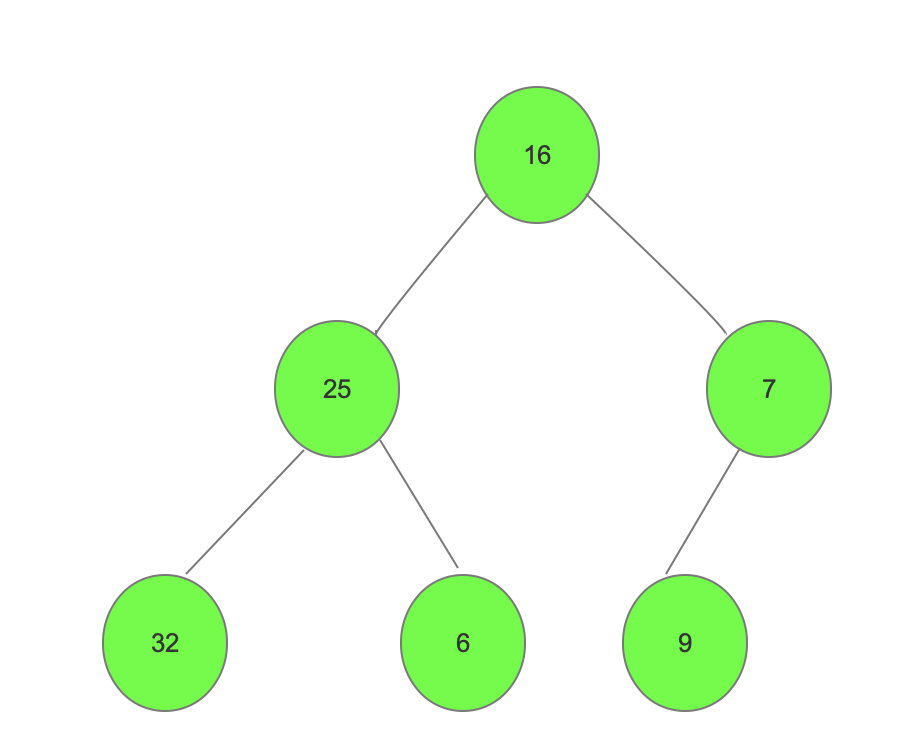3、步骤：
1、初始化一个堆型数据结构，调整堆结构，调整为大顶堆（从最后一个非叶子节点进行调整7比9小，交换7与9 -》32比25小，交换32和25-》16与32比较小，则32与16交换位置，16和25比较小，交换16和25的位置，形成了初始堆）。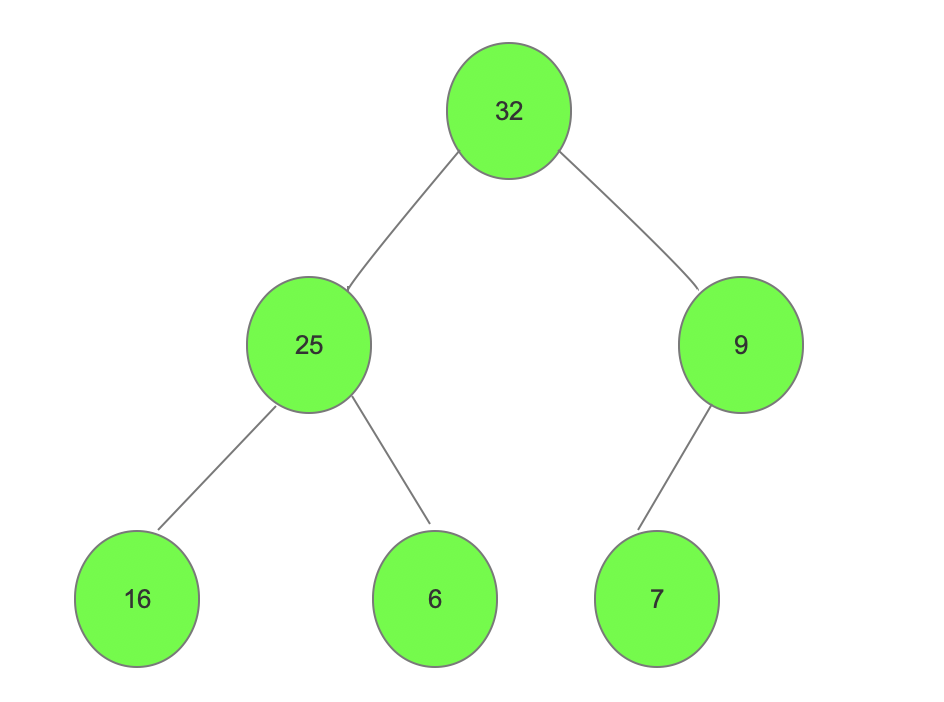2、将首未进行交换(将32与7进行交换)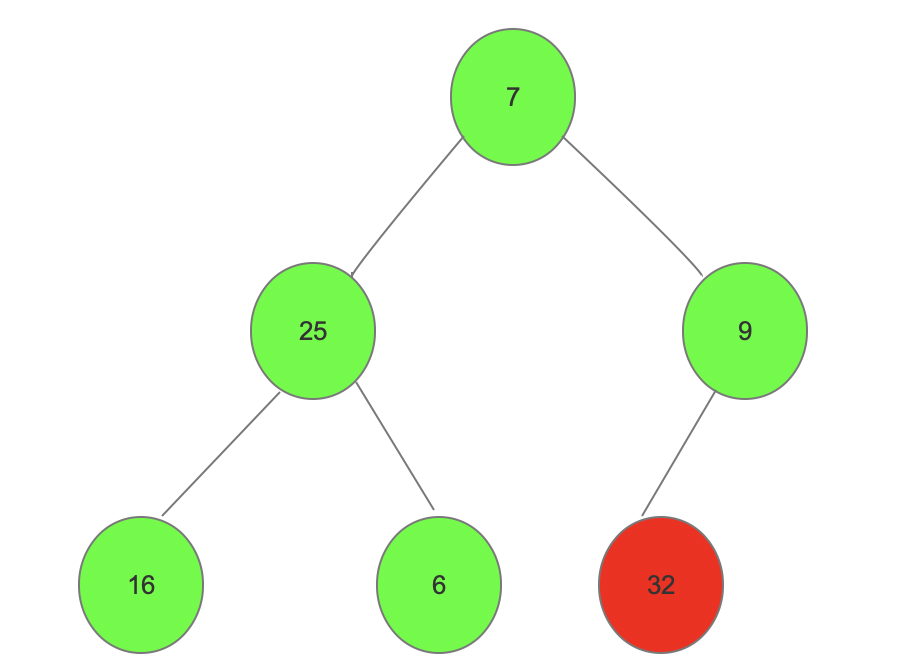3、将交换完的堆（7在最顶端不符合大顶堆），继续调整为大顶堆。并将首与倒第二个进行交换，然后以此类推直到根节点（建堆、交换、建堆。。。。直到要与根节点进行交换）

4、代码
4.1大顶堆排序
public class HeapSort1 {

public static void main(String[] args) {
int[] a = new int[]{16, 25, 7, 32, 6, 9};
heapSort(a);
System.out.println(Arrays.toString(a));
}

/**
* 构造大顶堆
* @param arr 待调整数组
* @param size 调整多少
* @param index 调整哪一个 最后一个叶子节点的父节点开始调整
*/
public static void maxHeap(int arr[], int size, int index) {

//左子节点
int leftNode = 2 * index + 1;
//右子节点
int rightNode = 2 * index + 2;

int max = index;//假设自己最大

//分别比较左右叶子节点找出最大
if(leftNode < size && arr[leftNode] > arr[max]) {//如果左侧叶子节点大于max则将最大位置换成leftNode并且递归需要限定范围为数组长度，
max = leftNode;//将最大位置改为左子节点
}

if(rightNode < size && arr[rightNode] > arr[max]) {//如果左侧叶子节点大于max则将最大位置换成rightNode
max = rightNode;//将最大位置改为右子节点
}

//如果不相等就需要交换
if(max != index) {
int tem = arr[index];
arr[index] = arr[max];
arr[max] = tem;
//如果下边还有叶子节点并且破坏了原有的堆。需要重新调整
maxHeap(arr, size, max);//位置为刚才改动的位置；
}
}

/**
* 需要将最大的顶部与最后一个交换
* @param arr
*/
public static void heapSort(int arr[]) {
int start = (arr.length - 1)/2;//开始位置最后一个非叶子节点，最后一个叶子节点的父节点
for(int i = start; i>=0; i--) {
maxHeap(arr, arr.length, i);
}

//最后一个跟第一个进行调整
for(int i = arr.length-1; i>0; i--) {//因为数组从零开始的，所以最后一个是数组长度减一
int temp = arr;//最前面的一个
arr = arr[i];//最后一个
arr[i] = temp;
//调整后再进行大顶堆调整
maxHeap(arr, i, 0);
}
}
}

4.2 小顶堆排序
public class HeapSortMin {
public static void main(String[] args) {
int[] a = new int[]{16, 25, 7, 32, 6, 9};
heapSort(a);//小顶堆
System.out.println(Arrays.toString(a));
}

/**
* 构造小顶堆
* @param arr 待调整数组
* @param size 调整多少
* @param index 调整哪一个 最后一个叶子节点的父节点开始调整
*/
public static void minHeap(int arr[], int size, int index) {

//左子节点
int leftNode = 2 * index + 1;
//右子节点
int rightNode = 2 * index + 2;

int min = index;//假设自己最小

//分别比较左右叶子节点找出最小
if(leftNode < size && arr[leftNode] < arr[min]) {//如果左侧叶子节点小于min则将最小位置换成leftNode并且递归需要限定范围为数组长度，
min = leftNode;//将最小位置改为左子节点
}

if(rightNode < size && arr[rightNode] < arr[min]) {//如果左侧叶子节点小于min则将最小位置换成rightNode
min = rightNode;//将最小位置改为右子节点
}

//如果不相等就需要交换
if(min != index) {
int tem = arr[index];
arr[index] = arr[min];
arr[min] = tem;
//如果下边还有叶子节点并且破坏了原有的堆。需要重新调整
minHeap(arr, size, min);//位置为刚才改动的位置；
}
}

/**
* 需要将最小的顶部与最后一个交换
* @param arr
*/
public static void heapSort(int arr[]) {
int start = (arr.length - 1)/2;//开始位置最后一个非叶子节点，最后一个叶子节点的父节点
for(int i = start; i>=0; i--) {
minHeap(arr, arr.length, i);
}

//最后一个跟第一个进行调整
for(int i = arr.length-1; i > 0; i--) {
int temp = arr;//最前面的一个
arr = arr[i];//最后一个
arr[i] = temp;
//调整后再进行小顶堆调整
minHeap(arr, i, 0);
}
}
}


5、实际应用（将上述堆排序代码进行改造，对方法heapSort进行改造）
5.1、从N个元素中查找最大的a个元素。也就是传说中的topK问题。
public class HeapSort2 {
public static void main(String[] args) {
int[] a = new int[]{16, 25, 7, 32, 6, 9};
int b [] = heapSort(a, 3);
System.out.println(Arrays.toString(b));
}

/**
* 构造大顶堆
* @param arr 待调整数组
* @param size 调整多少
* @param index 调整哪一个 最后一个叶子节点的父节点开始调整
*/
public static void maxHeap(int arr[], int size, int index) {

//左子节点
int leftNode = 2 * index + 1;
//右子节点
int rightNode = 2 * index + 2;

int max = index;//假设自己最大

//分别比较左右叶子节点找出最大
if(leftNode < size && arr[leftNode] > arr[max]) {//如果左侧叶子节点大于max则将最大位置换成leftNode并且递归需要限定范围为数组长度，
max = leftNode;//将最大位置改为左子节点
}

if(rightNode < size && arr[rightNode] > arr[max]) {//如果左侧叶子节点大于max则将最大位置换成rightNode
max = rightNode;//将最大位置改为右子节点
}

//如果不相等就需要交换
if(max != index) {
int tem = arr[index];
arr[index] = arr[max];
arr[max] = tem;
//如果下边还有叶子节点并且破坏了原有的堆。需要重新调整
maxHeap(arr, size, max);//位置为刚才改动的位置；
}
}

/**
* 需要将最大的顶部与最后一个交换
* @param arr
*/
public static int[] heapSort(int arr[], int a) {
int start = (arr.length - 1)/2;//开始位置最后一个非叶子节点，最后一个叶子节点的父节点
for(int i = start; i>=0; i--) {
maxHeap(arr, arr.length, i);
}
int count = 0;
//最后一个跟第一个进行调整
for(int i = arr.length-1; i > 0 && count < a; i--) {
int temp = arr;//最前面的一个
arr = arr[i];//最后一个
arr[i] = temp;
//调整后再进行大顶堆调整
maxHeap(arr, i, 0);
count++;
}
int[] result = new int[a];//定义一个新数组，长度为a
//其中：src表示源数组，srcPos表示源数组要复制的起始位置，desc表示目标数组，length表示要复制的长度。
System.arraycopy(arr, arr.length - a, result, 0, a);//将旧数组arr.length-a的位置到末尾进行复制到新数组中
return result;//返回值为所求最大a个元素
}
}

5.2、同时快速得到最大的n个元素和最小的n个元素倒叙
public class HeapSort2 {
public static void main(String[] args) {
int[] a = new int[]{16, 25, 7, 32, 6, 9};
int n = 3;
Map<String, Object> map = getMaxAndMinArray(a, n);
System.out.println("max n:"+Arrays.toString((int[]) map.get("max")));
System.out.println("min n:"+Arrays.toString((int[]) map.get("min")));
}

public static Map<String, Object> getMaxAndMinArray(int arr[], int n) {
int max[] = heapSort(arr, n, true);
int min[] = heapSort(arr, n, false);//最小堆写法
Map<String, Object> map = new LinkedHashMap<>();
map.put("max", max);
map.put("min", min);
return map;
}

/**
* 构造大、小顶堆
* @param arr 待调整数组
* @param size 调整多少
* @param index 调整哪一个 最后一个叶子节点的父节点开始调整
*/
public static void maxHeap(int arr[], int size, int index, Boolean isMax) {

//左子节点
int leftNode = 2 * index + 1;
//右子节点
int rightNode = 2 * index + 2;

int max = index;//假设自己最大、小
if(isMax) {
if(leftNode < size && arr[leftNode] > arr[max]) {//如果左侧叶子节点大于max则将最大位置换成leftNode并且递归需要限定范围为数组长度，
max = leftNode;//将最大位置改为左子节点
}
if(rightNode < size && arr[rightNode] > arr[max]) {//如果左侧叶子节点大于max则将最大位置换成rightNode
max = rightNode;//将最大位置改为右子节点
}
}else {
if(leftNode < size && arr[leftNode] < arr[max]) {//如果左侧叶子节点小于max则将最小位置换成leftNode并且递归需要限定范围为数组长度，
max = leftNode;//将最小位置改为左子节点
}
if(rightNode < size && arr[rightNode] < arr[max]) {//如果左侧叶子节点小于max则将最小位置换成rightNode
max = rightNode;//将最小位置改为右子节点
}
}
//如果不相等就需要交换
if(max != index) {
int tem = arr[index];
arr[index] = arr[max];
arr[max] = tem;
//如果下边还有叶子节点并且破坏了原有的堆。需要重新调整
maxHeap(arr, size, max, isMax);//位置为刚才改动的位置；
}
}

/**
* 需要将最大、最小的顶部与最后一个交换
* @param arr
*/
public static int[] heapSort(int arr[], int a, Boolean isMax) {
int start = (arr.length - 1)/2;//开始位置最后一个非叶子节点，最后一个叶子节点的父节点
for(int i = start; i>=0; i--) {
maxHeap(arr, arr.length, i, isMax);
}
int count = 0;
//最后一个跟第一个进行调整
for(int i = arr.length-1; i > 0 && count < a; i--) {
int temp = arr;//最前面的一个
arr = arr[i];//最后一个
arr[i] = temp;
//调整后再进行大、小顶堆调整
maxHeap(arr, i, 0, isMax);
count++;
}
int[] result = new int[a];
System.arraycopy(arr, arr.length - a, result, 0, a);
return result;
}
}


6、小顶堆删除代码
删除定义（弹出最小值）：小顶堆删除一个元素：删除总是发生在根A处。最后一个元素被用来填补空缺位置，结果树被更新以恢复堆条件。（说的挺感人的，其实就是删除最小堆的第一个元素，然后将最后一个元素放到数组第一个的位置，然后再调换位置成功小顶堆。）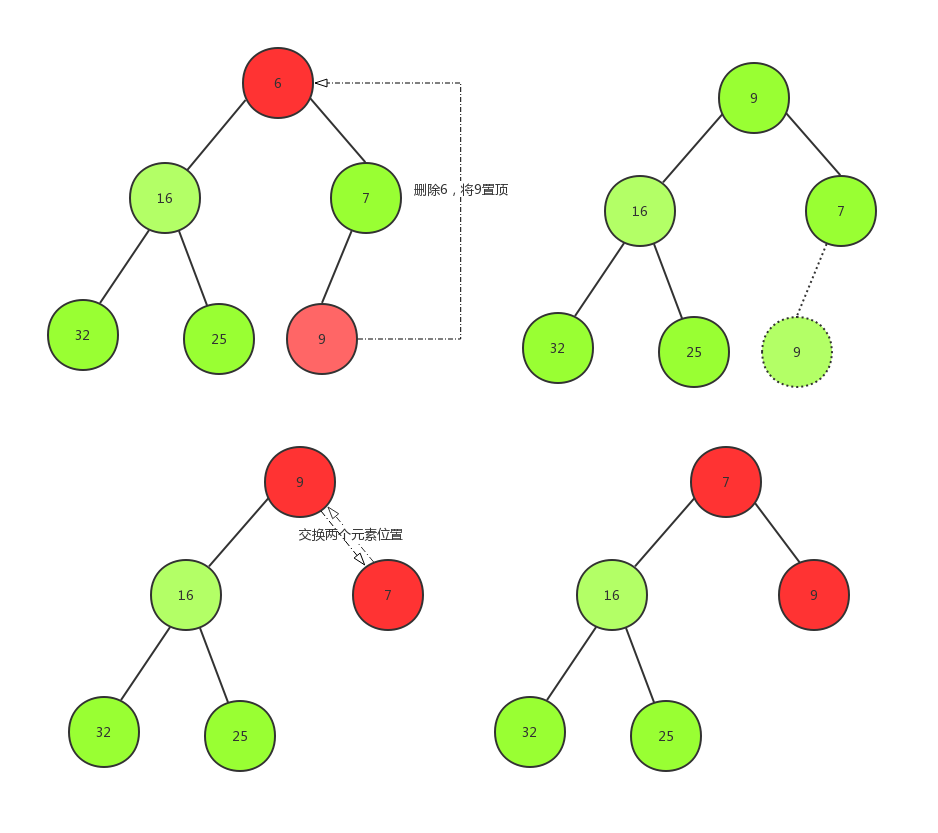可能有点low，没想到更改的解决办法。原理：1、删除顶点，2、将最后一个变为顶点 3、调整为小顶堆 4、数组长度减一
public class HeapSortMin {
public static void main(String[] args) {
int[] a = new int[]{16, 25, 7, 32, 6, 9};
int start = (a.length - 1)/2;//开始位置最后一个非叶子节点，最后一个叶子节点的父节点
System.out.println("未排序之前："+Arrays.toString(a));
minHeap(a, start);//小顶堆
System.out.println("小顶堆："+Arrays.toString(a));
//System.out.println("开始删除-----------");
//int[] b = delete(a, start);
//System.out.println("删除后小顶堆："+Arrays.toString(b));

System.out.println("开始插入-----------X");
int[] c = insert(a, start, 1);
System.out.println("插入后小顶堆："+Arrays.toString(c));
}

/**
* 构造小顶堆
* @param arr 待调整数组
* @param size 调整多少
* @param index 调整哪一个 最后一个叶子节点的父节点开始调整
*/
public static void minHeap(int arr[], int size, int index) {

//左子节点
int leftNode = 2 * index + 1;
//右子节点
int rightNode = 2 * index + 2;

int min = index;//假设自己最小

//分别比较左右叶子节点找出最小
if(leftNode < size && arr[leftNode] < arr[min]) {//如果左侧叶子节点小于min则将最大位置换成leftNode并且递归需要限定范围为数组长度，
min = leftNode;//将最小位置改为左子节点
}

if(rightNode < size && arr[rightNode] < arr[min]) {//如果左侧叶子节点小于min则将最小位置换成rightNode
min = rightNode;//将最小位置改为右子节点
}

//如果不相等就需要交换
if(min != index) {
int tem = arr[index];
arr[index] = arr[min];
arr[min] = tem;
//如果下边还有叶子节点并且破坏了原有的堆。需要重新调整
minHeap(arr, size, min);//位置为刚才改动的位置；
}
}

/**构造小顶堆
* @param arr
*/
public static void minHeap(int arr[], int start) {
for(int i = start; i>=0; i--) {
minHeap(arr, arr.length, i);
}
}

public static int[] delete(int[] arr, int start) {
int[] a = new int[arr.length - 1];
//现在开始是个小顶堆，1、删除顶点，2、将最后一个变为顶点 3、调整为小顶堆 4、数组长度减一
arr = arr[arr.length - 1];
arr[arr.length - 1] = -1;
for(int i = start; i >= 0; i--) {
minHeap(arr, arr.length - 1, i);//最后一个不进行小顶堆交换，因为是负一，比较无意义，最后要删除的
}
for(int i = 0; i < arr.length - 1 ;i++) {//获取除了最后一个的arr数组的所有元素
a[i] = arr[i];
}
return a;
}

/**
* 插入
* @param arr
* @param start 开始位置
* @param x 待插入的新值
* @return
*/
public static int[] insert(int arr[], int start, int x) {
//1、创建一个新数组长度比arr大一2、复制值到新的数组里面3、最后一位是要插入的补上去，4、交换位置为小顶堆结构
int[] a = new int[arr.length + 1];//扩容1
for(int i = 0; i <= arr.length - 1; i++) {
a[i] = arr[i];
}
a[arr.length] = x;
for(int i = start; i >= 0; i--) {
minHeap(a, a.length , i);
}
return a;
}
}

程序结果图：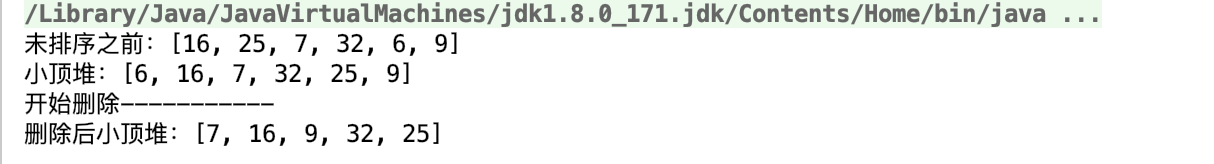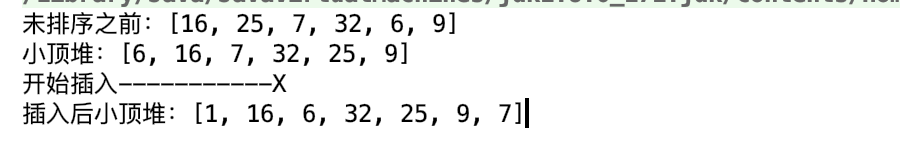7、小顶堆插入图解
小顶堆的插入是在堆尾部处插入。
逻辑是：1、创建一个新数组长度比arr大一2、复制值到新的数组里面3、最后一位是要插入的补上去，4、交换位置为小顶堆结构
代码在上边删除代码之下显示。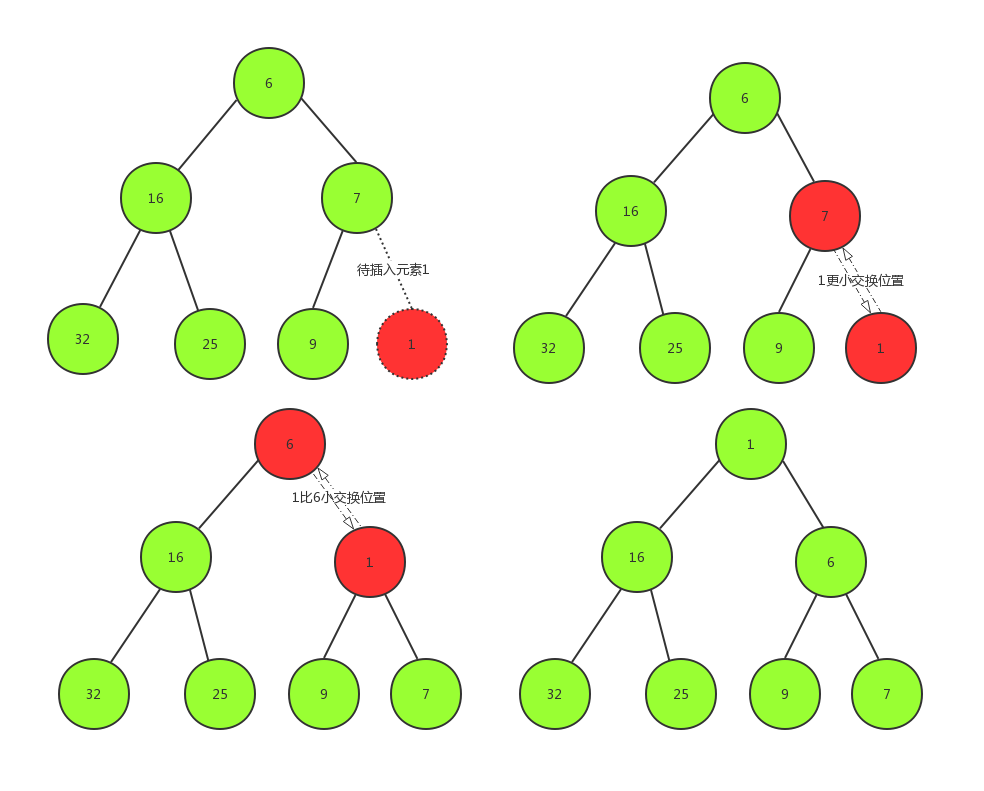8、复杂度 未完待续。。。


展开全文堆排序 大顶堆
• ## C++大顶堆和小顶堆

千次阅读 2020-11-24 09:04:59
C++大顶堆和小顶堆原理大顶堆小顶堆大顶堆和小顶堆对比图大顶堆和小顶堆的实现代码 原理   堆数据结构是一种数组对象，它可以被视为一颗完全二叉树结构（或者也有可能是满二叉树） 大顶堆   根结点（亦称为堆顶...


C++大顶堆和小顶堆
原理大顶堆小顶堆大顶堆和小顶堆对比图大顶堆和小顶堆的实现代码
vector和push_heap、pop_heap实现堆建堆调整堆
priority_queue实现堆简述模板参数成员函数大顶堆和小顶堆

原理
堆数据结构是一种数组对象，它可以被视为一颗完全二叉树结构（或者也有可能是满二叉树）
大顶堆
根结点（亦称为堆顶）的关键字是堆里所有结点关键字中最大者，称为大顶堆。大根堆要求根节点的关键字既大于或等于左子树的关键字值，又大于或等于右子树的关键字值。
小顶堆
根结点（亦称为堆顶）的关键字是堆里所有结点关键字中最小者，称为小顶堆。小根堆要求根节点的关键字既小于或等于左子树的关键字值，又小于或等于右子树的关键字值。
大顶堆和小顶堆对比图大顶堆和小顶堆的实现代码
heap.h
#pragma once
#include<iostream>
#include<assert.h>
#include<vector>
using namespace std;

template<class T>
struct Less
{
bool operator()(const T& left, const T& right) const
{
return left < right;
}
};

template<class T>
struct Greater
{
bool operator()(const T& left, const T& right) const
{
return left > right;
}
};

template<class T, class Compare = Less<T>>
class Heap
{
public:
Heap()//无参的构造函数（系统不会给无参构造函数），开始堆是空的不需要做什么事
{}
Heap(T* a, size_t n)
{
_a.reserve(n);//开空间
for (size_t i = 0; i < n; ++i)
{
_a.push_back(a[i]);

}
//建堆,找最后一个非叶子节点
for (int i = (_a.size() - 2) / 2; i >= 0; --i)//不用size_t，因为i在这可能等于0，用size_t会死循环
{
}
}
//向下调整
{
Compare com;
int parent = root;
size_t child = parent * 2 + 1;//默认为左孩子
while (child < _a.size())
{
//选出小孩子
//if (child+1 > _a.size() && _a[child + 1]< _a[child])
if (child + 1 < _a.size() && com(_a[child + 1], _a[child]))
{
++child;
}

//if (_a[child] < _a[parent])
if (com(_a[child], _a[parent]))
{
swap(_a[child], _a[parent]);//交换值
parent = child;
child = parent * 2 + 1;
}
else
{
break;
}
}
}
//向上调整
{
Compare com;
int parent = (child - 1) / 2;
while (parent >= 0)
{
//if (_a[child] < _a[parent])
if (com(_a[child], _a[parent]))
{
swap(_a[parent], _a[child]);
child = parent;
parent = (child - 1) / 2;
}
else
{
break;
}
}

}
//最后插入
void Push(const T&x)
{
_a.push_back(x);
}
//删除最大数
void Pop()
{
assert(!_a.empty());
swap(_a, _a[_a.size() - 1]);
_a.pop_back();

}
//取顶元素
T& Top()
{
assert(!_a.empty());
return _a;
}
size_t Size()
{
return _a.size();
}

bool Empty()
{
return _a.empty();
}

private:
vector<T> _a;

};

main.cpp
#include <iostream>
#include "heap.h"
using namespace std;

int main()
{
int a[] = { 10,11,13,12,16,18,15,17,14,19 };
// Heap<int,Greater<int>> hp1(a,sizeof(a)/sizeof(a)); 最大堆
// Heap<int,Less<int>> hp1(a,sizeof(a)/sizeof(a)); 最小堆
Heap<int> hp1(a, sizeof(a) / sizeof(a)); // 缺省，最小堆
hp1.Push(15);
system("pause");
return 0;
}

vector和push_heap、pop_heap实现堆
建堆
vector<int> nums = {9, 6, 2, 4, 7, 0, 1, 8, 3, 5};

如何使用nums构建最大堆
make_heap(nums.begin(), nums.end());
//或
make_heap(nums.begin(), nums.end(), less<int>());

如何使用nums构建最小堆
make_heap(nums.begin(), nums.end(), greater<int>());

调整堆
当使用上述的make_heap()建完堆后，如果vector使用push_back()插入数据或pop_back()删除数据后，会破坏最大堆/最小堆的性质，所以需要调整堆，常用push_heap()和pop_heap()两个方法。 1、push_heap()用法是，vector先push_back()，后push_heap()
nums.push_back(10);
push_heap(nums.begin(), nums.end(), less<int>());

2、pop_heap()用法是，先pop_heap()，vector后pop_back()
pop_heap(nums.begin(), nums.end(), less<int>());
nums.pop_back();

为什么pop_heap()的用法要反过来呢？ 要从我们的目的来考虑，使用pop_heap()的绝大部分目的是要把堆顶元素pop出堆中，因为它最大或最小。如果先用vector的pop_back()，它删除的不是堆顶元素（nums），而是vector的最后一个元素。可见这不是我们想要的结果：对于最大堆，最后一个元素既不是最大，也不一定是最小；对于最小堆，最后一个元素既不是最小，也不一定是最大。pop出来没有意义。 观察pop_heap()对堆做了什么？ pop_heap()把堆顶元素放到了最后一位，然后对它前面的数字重建了堆。这样一来只要再使用pop_back()把最后一位元素删除，就得到了新的堆。
priority_queue实现堆
priority_queue 对于这个模板类priority_queue，它是STL所提供的一个非常有效的容器。 作为队列的一个延伸，优先队列包含在头文件 中。
简述
优先队列时一种比较重要的数据结构，它是有二项队列编写而成的，可以以O(log n) 的效率查找一个队列中的最大值或者最小值，其中是最大值还是最小值是根据创建的优先队列的性质来决定的。
模板参数
优先队列有三个参数，其声明形式为：
priority_queue< type, container, function >

这三个参数，后面两个可以省略，第一个不可以。其中： **type：**数据类型； **container：**实现优先队列的底层容器； **function：**元素之间的比较方式； 对于container，要求必须是数组形式实现的容器，例如vector、deque，而不能使list。 在STL中，默认情况下（不加后面两个参数）是以vector为容器，以 operator< 为比较方式，所以在只使用第一个参数时，优先队列默认是一个最大堆，每次输出的堆顶元素是此时堆中的最大元素。
成员函数
假设type类型为int，则：
bool empty() const 返回值为true，说明队列为空；int size() const 返回优先队列中元素的数量；void pop() 删除队列顶部的元素，也即根节点；int top() 返回队列中的顶部元素，但不删除该元素；void push(int arg) 将元素arg插入到队列之中。
大顶堆和小顶堆
#include <functional>

//构造一个空的优先队列（此优先队列默认为大顶堆）
priority_queue<int> big_heap;
//另一种构建大顶堆的方法
priority_queue<int,vector<int>,less<int> > big_heap2;

//构造一个空的优先队列,此优先队列是一个小顶堆
priority_queue<int,vector<int>,greater<int> > small_heap;

展开全文• 小顶堆 每个结点的值都小于或等于其左右孩子结点的值 对比图 实现代码 public class HeapNode{ private int size;//堆大小 private int[] heap;//保存堆数组 //初始化堆 public HeapNode(int n) { heap = new...java 数据结构 算法
• 可以看到，本质上，大顶堆是小顶堆调了 Comparator 进行了比较，实现匿名内部类 提到匿名内部类，就不得不提 lambda表达式，上面的代码就可以写成下面这样了 PriorityQueue<Integer> maxheap = new PriorityQueue(...java
• ## 堆排序（浅谈大顶堆与小顶堆）

万次阅读 多人点赞 2019-09-14 15:20:11
堆是一种非线性结构，（本篇随笔主要分析堆的数组实现）可以把堆看作一个数组，也可以被看作一个完全二叉树，通俗来讲堆其实就是利用完全二叉树的结构来维护的一维数组，按照堆的特点可以把堆分为大顶堆和小顶堆。...
• 按照堆的特点可以把堆分为大顶堆和小顶堆 大顶堆：每个结点的值都大于或等于其左右孩子结点的值 小顶堆：每个结点的值都小于或等于其左右孩子结点的值 使用堆的原因？ 如果仅仅是需要得到一个有序的序列，使用排序就...
• 文章目录 priority_queue 基本用法 大顶堆 小顶堆 vector 排序 优先队列自定义排序 priority_queue 基本用法 头文件 priority_queue, container, function> type 类型 container：实现优先队列的底层容器（缺省） ...
• 小顶堆：任一节点小于左右孩子节点值（大顶堆则是打于，本文以小顶堆为例） 堆在概念上是完全二叉树，由于完全二叉树父子节点顺序间的特殊代数关系： // 对于节点 n, 其左孩子 l，右孩子 r，父节点p，分别代表...java 数据结构 算法 排序算法
• 基础数据结构 ...大顶堆和小顶堆 我们知道大顶推满足的条件是每一个父节点都比子节点大。 那么我们应该如何通过调换节点的位置来构建这样的数据结构呢？ 我们取这个树的一个片段，假设数据为2，1，3。那.
• 一、大顶堆和小顶堆的原理 1、大顶堆 根结点（亦称为堆顶）的关键字是堆里所有结点关键字中最大者，称为大顶堆。大根堆要求根节点的关键字既大于或等于左子树的关键字值，又大于或等于右子树的关键字值。 2、...
• 【一】简介 最小堆是一棵完全二叉树，非叶子结点的值不大于左孩子和右孩子的值。本文以图解的方式，说明最小堆的构建、插入、删除的过程...根据性质，的数字往上移动；至此，第1次调整完成。 注意，被调整的节点...数据结构 插入 删除
• 大顶堆和小顶堆的理论是相同的，实现上就只有符号的变化。 堆的含义 堆是类似与完全二叉树的结构（注意可能不是满二叉树）， 堆的结构是要使得每个根节点的元素大于（小与）子节点元素，左右节点是没有要求的。 ...堆排序 大顶堆 heap
• 思路如下： 创建一个大顶堆和一个小顶堆，大顶堆的堆顶元素比小顶堆的堆顶元素更小，大顶堆维护 99% 的请求时间，小顶堆维护 1% 的请求时间 每产生一个元素(请求时间)，如果它比大顶堆的堆顶元素小，则将其放入到大...
• 大顶堆和小顶堆是什么？ 概述：大顶堆和小顶堆的概念都是从堆的概念引申而来，对于n个元素的关键字序列{K1， K2，…，Kn}， 当且仅当满足下列关系时称其为堆，其中2i和2i+1要求不大于n。 若将此序列对应的一维数组...二叉树
• ​ 堆是一种数据结构，实质是利用完全二叉树结构来维护的一维数组，按照堆的特点可以把堆分为大顶堆和小顶堆。 大顶堆：每个结点的值都大于或等于其左右孩子结点的值； 小顶堆：每个结点的值都小于或等于其左右孩子...队列 java 算法
• java - 算法 - 大顶堆、小顶堆 排序 一、完全二叉树的数组表示形式特性 最后一个父节点下标为 (len/2)-1 若当前节点的下标为i 父节点的下标为 (i-1)/2 左子节点的下标为 (i*2)+1 或 (i<<1)+1 右子节点的下标...
• 大顶堆升序、小顶堆降序的堆排序（以Java描述为例版本） 一、定义 堆是具有以下性质的完全二叉树：每个结点的值都大于或等于其左右孩子结点的值，称为大顶堆；或者每个结点的值都小于或等于其左右孩子结点的值，...二叉树 数据结构 java
• } 使用大顶堆（小顶堆）解决TopK问题 拿小顶堆举例来说，堆顶就是当前K个中最小的。如果最大的前K个元素都在这个堆中，那堆顶就是第K大的那个元素。 初始化堆，堆的大小为k。（直接拿数组前K个元素初始就行） 从arr...
• 由上述性质可知大顶堆的堆顶的关键字肯定是所有关键字中最大的，小顶堆的堆顶的关键字是所有关键字中最小的。 堆排序思想： 利用大顶堆 ( 小顶堆 ) 堆顶记录的是最大关键字 ( 最小关键字 ) 这一特性，使得每次从无序...
• 二叉堆就是一颗二叉树，是一颗完全二叉树，最直观表现一个二叉树左边最多比右边深 1 层，二叉堆我们常常讨论的就是大顶堆和小顶堆，其中大顶堆根结点最大，左右节点依次递归，小顶堆类似 二叉堆算是一种比较重要的...二叉堆 heap 数据结构 算法
• 首先明确堆是一个完全二叉树，小顶堆指根结点的值小于或等于左右子节点的值，大顶堆指根结点的值都大于或等于左右子节点的值 关于大小顶堆的建立更详细的介绍 #include<iostream> #include<cstdio> #...
• 首先我们需要构建一个小顶堆 我们可以用PriorityQueue这个优先队列,它给我们从小到大排序好了的,至于什么是小顶堆可以去看看堆和数的概念. PriorityQueue pq = new PriorityQueue<>(); 我们需要对小顶堆的堆...java 堆排序 算法
• 构造大顶堆（Javascript实现） 大顶堆的概念：每个结点的值都大于或等于其左右孩子结点的值 给定一个序列，按照序列的顺序先依次组成一个完全二叉树，然后再将不稳定的节点的值进行处理，形成大顶堆 //构建大顶堆 ...数据结构 javascript
• Python小顶堆的实现 以前都是用的heapq，这次面试碰到让自己实现一个堆得到top k的元素。抛砖引玉，希望各位提些意见指正 class Heap: def __init__(self, k): self.val = [] self.k = k def get_left(self, ...python
• 大顶堆小顶堆的意义几乎一致，所以只举小顶堆的例子。 堆定义 堆实际上是一棵完全二叉树，其任何一非叶节点满足性质： Key[i]<=key[2i+1]&&Key[i]<=key[2i+2]（小顶堆）或者：Key[i]>=Key[2i+1]&...
• C++中的堆是大顶堆（大根堆），一般也是常用的一种堆，但是py中还是选择了实现一个小顶堆。 内置方法 创建堆： 如果是用过counter的，可能感觉上是差不多的，就是对一个可迭代序列调用方法即可 heapify(iterable) ...python heapq库...